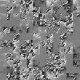author Amos RobinsonWed, 28 Nov 2012 23:19:42 +0000 (10:19 +1100) committer Amos RobinsonWed, 28 Nov 2012 23:19:42 +0000 (10:19 +1100)
 dph-examples/examples/spectral/Rotation/MainGloss [new file with mode: 0755] patch | blob dph-examples/examples/spectral/Rotation/MainGloss.hs [new file with mode: 0644] patch | blob dph-examples/examples/spectral/Rotation/Solver.hs [new file with mode: 0644] patch | blob dph-examples/examples/spectral/Rotation/Vector.hs [new file with mode: 0644] patch | blob dph-examples/examples/spectral/Rotation/Vectorised.hs [new file with mode: 0644] patch | blob

diff --git a/dph-examples/examples/spectral/Rotation/MainGloss b/dph-examples/examples/spectral/Rotation/MainGloss
new file mode 100755 (executable)
index 0000000..cf15d51
Binary files /dev/null and b/dph-examples/examples/spectral/Rotation/MainGloss differ
diff --git a/dph-examples/examples/spectral/Rotation/MainGloss.hs b/dph-examples/examples/spectral/Rotation/MainGloss.hs
new file mode 100644 (file)
index 0000000..54cc6fa
--- /dev/null
@@ -0,0 +1,61 @@
+{-# LANGUAGE ParallelListComp, BangPatterns #-}
+
+import Solver
+
+import Graphics.Gloss
+
+import System.Environment
+import Data.Maybe
+import qualified Data.Vector                    as V
+import qualified Data.Vector.Unboxed            as VU
+
+
+main :: IO ()
+main
+ = do   args    <- getArgs
+        mainWithArgs args
+
+
+mainWithArgs :: [String] -> IO ()
+mainWithArgs [solverName,depthStr]
+ = let  -- The solver we're using to calculate the acclerations.
+        solver      = fromMaybe (error \$ unlines
+                                        [ "unknown solver " ++ show solverName
+                                        , "choose one of "  ++ (show \$ map fst solvers) ])
+                        \$ lookup solverName solvers
+
+        depth = read depthStr
+    in  mainGloss depth solver 400
+
+mainWithArgs [solverName] = mainWithArgs [solverName,"4"]
+
+mainWithArgs _ = putStrLn "Usage: rotations <vector|vectorised> <depth>"
+
+
+-- | Run the simulation in a gloss window.
+mainGloss
+        :: Int          -- ^ Depth
+        -> Solver       -- ^ Fn to calculate accels of each point.
+        -> Int          -- ^ Size of window.
+        -> IO ()
+
+mainGloss depth solver windowSize
+ = let  draw t
+         = let  pts = solver depth (realToFrac t)
+           in   Color white \$ Pictures \$ map drawPoint \$ VU.toList pts
+
+   in   animate
+                (InWindow  "Silly"                    -- window name
+                           (windowSize, windowSize)   -- window size
+                           (10, 10))                  -- window position
+                black                                 -- background color
+                draw                                  -- fn to convert a world to a picture
+
+
+
+pointSize = 4
+
+drawPoint :: (Double, Double) -> Picture
+drawPoint (x, y)
+       = Translate (realToFrac x * 50) (realToFrac y * 50)
+       \$ ThickCircle (pointSize / 2) pointSize
diff --git a/dph-examples/examples/spectral/Rotation/Solver.hs b/dph-examples/examples/spectral/Rotation/Solver.hs
new file mode 100644 (file)
index 0000000..08560f1
--- /dev/null
@@ -0,0 +1,30 @@
+
+-- | Wrappers for the various solvers.
+module Solver
+       ( Solver
+       , solvers)
+where
+import qualified Vector                    as SV
+import qualified Vectorised            as SPA
+
+import qualified Data.Vector                   as V
+import qualified Data.Vector.Unboxed           as VU
+
+import qualified Data.Array.Parallel   as P
+import qualified Data.Array.Parallel.PArray    as P
+
+type Solver    = Int -> Double -> VU.Vector (Double,Double)
+
+solvers :: [(String, Solver)]
+solvers
+ =     [ ("vectorised",                solver_spa)
+    , ("vector",                   SV.solveV)
+    ]
+
+-- | Nested Data Parallelism + Barnes-Hut algorithm.
+solver_spa     :: Solver
+solver_spa depth time
+ = let
+       pts'    = SPA.solvePA depth time
+   in  VU.fromList \$ P.toList pts'
+
diff --git a/dph-examples/examples/spectral/Rotation/Vector.hs b/dph-examples/examples/spectral/Rotation/Vector.hs
new file mode 100644 (file)
index 0000000..ff87550
--- /dev/null
@@ -0,0 +1,41 @@
+module Vector
+    (solveV
+    )
+where
+import qualified Data.Vector.Unboxed    as VU
+
+type Point = (Double,Double)
+
+{-# NOINLINE solveV #-}
+solveV
+    :: Int      -- ^ depth
+    -> Double   -- ^ time
+    -> VU.Vector Point
+solveV depth t
+ = let s p   = solveV' t depth p
+   in s 0 VU.++ s (pi/2) VU.++ s pi VU.++ s (3*pi / 2)
+
+solveV' :: Double -> Int -> Double -> VU.Vector Point
+solveV' t iG pG
+ = let
+       {-# INLINE l #-}
+       l         = fromIntegral iG
+       {-# INLINE p #-}
+       p         = pG
+       {-# INLINE f #-}
+       f         = fromIntegral iG / 20
+
+
+       {-# INLINE r' #-}
+       r'        = p + f*t
+       cos'      = cos r'
+       sin'      = sin r'
+       (px, py)  = (- l * sin', l * cos')
+
+       {-# INLINE pts #-}
+       pts       = VU.concatMap (\iG2 -> solveV' t (iG - 1) (fromIntegral iG2 / l * 2 * pi - pi)) (VU.enumFromN (1::Int) iG)
+       {-# INLINE pts' #-}
+       pts'      = VU.map (\(x,y) -> (x * cos' - y * sin' + px, x * sin' + y * cos' + py)) pts
+   in VU.singleton (px, py) VU.++ pts'
+
+
diff --git a/dph-examples/examples/spectral/Rotation/Vectorised.hs b/dph-examples/examples/spectral/Rotation/Vectorised.hs
new file mode 100644 (file)
index 0000000..a7a8535
--- /dev/null
@@ -0,0 +1,79 @@
+{-# LANGUAGE ParallelArrays, ParallelListComp #-}
+{-# OPTIONS -fvectorise #-}
+
+module Vectorised
+    (solvePA
+    , pi
+    , solveV2
+    )
+where
+import Data.Array.Parallel hiding ((+), (-), (*), (/))
+import Data.Array.Parallel.PArray
+import Data.Array.Parallel.Prelude.Bool
+import Data.Array.Parallel.Prelude.Double        as D hiding (pi)
+import qualified Data.Array.Parallel.Prelude.Int as I
+import qualified Prelude    as P
+
+{-
+data NodeV = NodeV Double Double Double [:NodeV:]
+-}
+
+pi = 3.1415926535
+type Point = (Double,Double)
+
+{-# NOINLINE solvePA #-}
+solvePA
+    :: Int      -- ^ depth
+    -> Double   -- ^ time
+    -> PArray Point
+solvePA depth t
+ = let s p   = solveV2 t depth p
+   in toPArrayP (s 0 +:+ s (pi/2) +:+ s pi +:+ s (3*pi / 2))
+
+solveV2 :: Double -> Int -> Double -> [:Point:]
+solveV2 t iG pG
+ = let
+       {-# INLINE l #-}
+       l         = fromInt iG
+       {-# INLINE p #-}
+       p         = pG
+       {-# INLINE f #-}
+       f         = fromInt iG / 20
+
+
+       {-# INLINE r' #-}
+       r'        = p + f*t
+       cos'      = cos r'
+       sin'      = sin r'
+       (px, py)  = (- l * sin', l * cos')
+
+       {-# INLINE pts #-}
+       pts       = concatP (mapP (\iG2 -> solveV2 t (iG I.- 1) (fromInt iG2 / l * 2 * pi - pi)) (I.enumFromToP 1 iG))
+       {-# INLINE pts' #-}
+       pts'      = mapP (\(x,y) -> (x * cos' - y * sin' + px, x * sin' + y * cos' + py)) pts
+   in singletonP (px, py) +:+ pts'
+
+
+{-
+mkNode :: Int -> Double -> NodeV
+mkNode i p
+ = let i' = fromInt i
+   in  NodeV i' p (i' / 20)
+       (mapP (\i2 -> mkNode (i I.- 1) (fromInt i2 / i' * 2 * pi - pi)) (I.enumFromToP 1 i))
+
+
+{-# INLINE solveV #-}
+solveV :: Double -> NodeV -> [:Point:]
+solveV t (NodeV l p f c)
+ = let r'        = p + f*t
+       cos'      = cos r'
+       sin'      = sin r'
+       rot (x,y) = (x * cos' - y * sin', x * sin' + y * cos')
+       (px, py)  = rot (0, l)
+       trn (x,y) = (x + px, y + py)
+
+       pts       = concatP (mapP (solveV t) c)
+       pts'      = mapP (\p -> trn (rot p)) pts
+   in singletonP (px, py) +:+ pts'
+
+-}